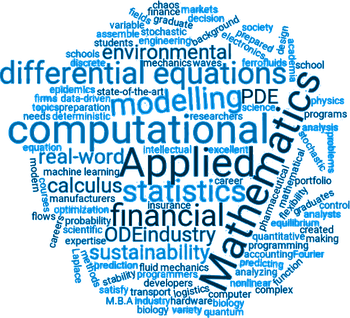# ResearchThe core group of AM2 members have expertises covering mathematical analysis, numerical analysis, applied probability, dynamical systems, multiscale modeling, high performance scientific computing, and numerical optimization.

Members are also directly involved in applications, among others in image processing, computer tomography, data science and artificial intelligence, models of visual cortex and brain inspired neural networks, biology, fluid dynamics, geosciences, finance.

Some of the principal research directions of applied mathematics pursued at AM2 are: predictive modeling and data science,  numerical analysis and scientific computing, including methods for deterministic and stochastic PDE, numerical inverse problems, image processing, geometrical design, stochastic modelling, probability theory, stochastic processes and equilibrium and nonequilibrium statistical mechanics; Nonlinear PDE, harmonic analysis, dynamical systems, ergodic theory and mathematical physics,  mathematical biology and ecology.